# High School Math : How to find the volume of a pyramid

## Example Questions

### Example Question #1 : Pyramids

What is the volume of a pyramid with a height ofand a square base with a side length of?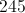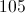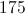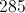Explanation:

To find the volume of a pyramid we must use the equation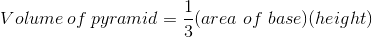We must first solve for the area of the square using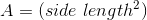We plug inand square it to get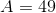We then plug our answer into the equation for the pyramid with the height to get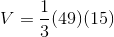We multiply the result to get our final answer for the volume of the pyramid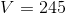.

### Example Question #1 : Pyramids

Find the volume of the following pyramid. Round the answer to the nearest integer.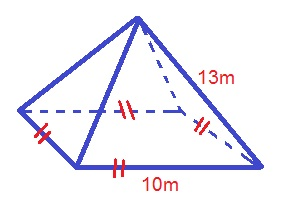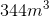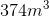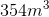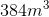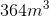Explanation:

The formula for the volume of a pyramid is: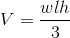where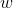is the width of the base,is the length of the base, andis the height of the pyramid.

In order to determine the height of the pyramid, you will need to use the Pythagoream Theorem to find the slant height: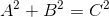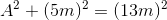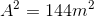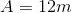Now we can use the slant height to find the pyramid height, once again using the Pythagoream Theorem: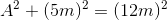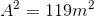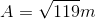Plugging in our values, we get: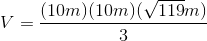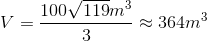### Example Question #2 : Pyramids

Find the volume of the following pyramid.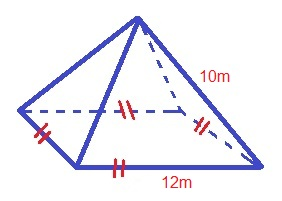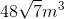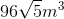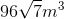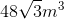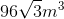Explanation:

The formula for the volume of a pyramid is: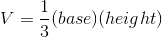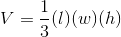Whereis the length of the base,is the width of the base, andis the height of the pyramid

Use the Pythagorean Theorem to find the length of the slant height: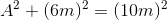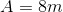Now, use the Pythagorean Theorem again to find the length of the height: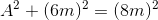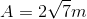Plugging in our values, we get: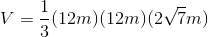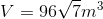### Example Question #1 : How To Find The Volume Of A Pyramid

What is the sum of the number of vertices, edges, and faces of a square pyramid?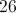Explanation:

A square pyramid has one square base and four triangular sides.

Vertices (where two or more edges come together):  5. There are four vertices on the base (one at each corner of the square) and a fifth at the top of the pyramid.

Edges (where two faces come together):  8. There are four edges on the base (one along each side) and four more along the sides of the triangular faces extending from the corners of the base to the top vertex.

Faces (planar surfaces):  5. The base is one face, and there are four triangular faces that form the top of the pyramid.

Total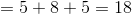### Example Question #5 : How To Find The Volume Of A Pyramid

An architect wants to make a square pyramid and fill it with 12,000 cubic feet of sand. If the base of the pyramid is 30 feet on each side, how tall does he need to make it?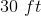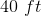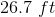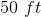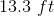Explanation:

Volume of Pyramid = 1/3 * Area of Base * Height

12,000 ft3 = 1/3 * 30ft * 30ft * H

12,000  = 300 * H

H = 12,000  / 300 = 40

H = 40 ft

### Example Question #1 : Pyramids

The volume of a 6-foot-tall square pyramid is 8 cubic feet. How long are the sides of the base?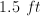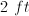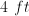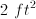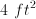Explanation:

Volume of a pyramid is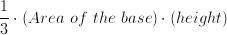Thus: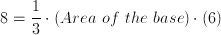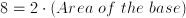Area of the base is.

Therefore, each side is.